Do you like the article?
Share it with others -

Use new possibilities of MetaTrader 5

# Movement continuation model - searching on the chart and execution statistics

30 November 2018, 12:45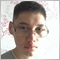0
7 322

### 1. Introduction

This article provides programmatic definition of one of the movement continuation models. The main idea is defining two waves — the main and the correction one. For extreme points, I apply fractals as well as "potential" fractals — extreme points that have not yet formed as fractals. Next, I will try to collect statistical data on the waves movement. The data will be uploaded to a CSV file.

### 2. Model description - general features

Movement continuation model described in the article consists of two waves: the main and the correction one. The model is schematically described in Figure 1. AB is the main wave, BC is the correction wave, while CD is the continuation of the movement towards the main trend.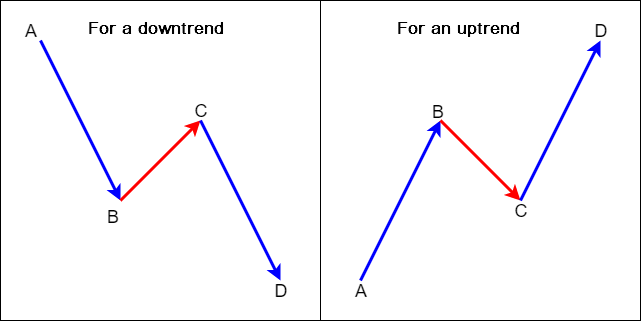Fig. 1. Movement continuation model

On the chart, this looks as follows: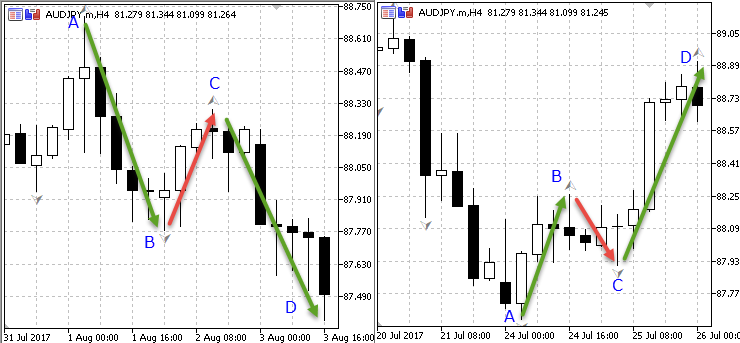Fig. 2. Movement continuation model on AUDJPY H4

### 3. Principles of the model recognition on the chart

The model recognition principles are described in table 1.

Table 1. Movement continuation model recognition principles in the context of trends

Model recognition principles for a downward trend  # Model recognition principles for an upward trend
1 The extremum bar is a bar having High/Low above/below the two High/Lows of the previous bars 1 The extremum bar is a bar having High/Low above/below the two High/Lows of the previous bars
2 A correction wave should always end with the presence of an upper extremum (point С - see Fig. 1 and Fig. 2) 2 The correction wave should always end with the presence of a lower extremum (point С - see Fig. 1 and Fig. 2)
3 The duration of the correction wave cannot be long and should be limited to several bars.  3 The duration of the correction wave cannot be long and should be limited to several bars.
4 High of the correction movement (point С - see Fig. 1 and Fig. 2) should be lower than High of the main movement (point A - see Fig. 1 and Fig. 2)  4 Low of the correction movement (point С - see Fig. 1 and Fig. 2) should be higher than Low of the main movement (point A - see Fig. 1 and Fig. 2)
5 Entry point timeliness principle - a position should be opened only at a certain moment of the entry point formation  5 Entry point timeliness principle - a position should be opened only at a certain moment of the entry point formation

### 1. Input parameters, OnInit() function and initial variable declaration

```//--- include the files
//--- object for conducting trading operations

Next, define input parameters:

```//--- input parameters
input ENUM_TIMEFRAMES base_tf;  //base period timeframe
input ENUM_TIMEFRAMES work_tf;  //working period timeframe
input double SummRisk=100;      //total risk per deal
input double sar_step=0.1;      //set parabolic step
input double maximum_step=0.11; //set parabolic maximum step
input bool TP_mode=true;        //allow setting take profit
input int M=2;                  //profit to risk ratio
input bool Breakeven_mode=true; //allow moving a position to a breakeven
input double breakeven=1;       //profit to stop loss ratio```

On the base period, the EA defines the entry direction, while the working period is used to define the entry point.

The program calculates the lot size depending on the total risk per deal.

The EA can also set take profit based on the specified profit to risk ratio (М parameter) and move a position to breakeven based on the specified profit to stop loss ratio (breakeven parameter).

After describing input parameters, declare the variables for the indicator handles and arrays for the base_tf and work_tf timeframes:

```//--- declare the variables for the indicator handles
int Fractal_base_tf,Fractal_work_tf;             //iFractals indicator handle
int Sar_base_tf,Sar_work_tf;                     //iSar indicator handle
//--- declare arrays for base_tf
double High_base_tf[],Low_base_tf[];             //arrays for storing the prices of High and Low bars
double Close_base_tf[],Open_base_tf[];           //arrays for storing the prices of Close and Open bars
datetime Time_base_tf[];                         //array for storing bar open time
double Sar_array_base_tf[];                      //array for storing iSar (Parabolic) indicator prices
double FractalDown_base_tf[],FractalUp_base_tf[];//array for storing iFractals indicator prices
//--- declare arrays for work_tf
double High_work_tf[],Low_work_tf[];
double Close_work_tf[],Open_work_tf[];
datetime Time_work_tf[];
double Sar_array_work_tf[];
double FractalDown_work_tf[],FractalUp_work_tf[];;```

The EA applies two indicators: fractals for defining part of extremums and Parabolic for maintaining position's trailing stop. I am also going to use Parabolic to define an entry point on the work_tf working timeframe.

Then receive the indicator handles in the OnInit() function and fill the arrays with initial data.

```int OnInit()
{
//--- get iSar indicator handle
Sar_base_tf=iSAR(Symbol(),base_tf,sar_step,maximum_step);
Sar_work_tf=iSAR(Symbol(),work_tf,sar_step,maximum_step);
//--- get iFractals indicator handles
Fractal_base_tf=iFractals(Symbol(),base_tf);
Fractal_work_tf=iFractals(Symbol(),work_tf);
//--- set the order of arrays as in the timeseries for base_tf
ArraySetAsSeries(High_base_tf,true);
ArraySetAsSeries(Low_base_tf,true);
ArraySetAsSeries(Close_base_tf,true);
ArraySetAsSeries(Open_base_tf,true);
ArraySetAsSeries(Time_base_tf,true);;
ArraySetAsSeries(Sar_array_base_tf,true);
ArraySetAsSeries(FractalDown_base_tf,true);
ArraySetAsSeries(FractalUp_base_tf,true);
//--- initial arrays filling for base_tf
CopyHigh(Symbol(),base_tf,0,1000,High_base_tf);
CopyLow(Symbol(),base_tf,0,1000,Low_base_tf);
CopyClose(Symbol(),base_tf,0,1000,Close_base_tf);
CopyOpen(Symbol(),base_tf,0,1000,Open_base_tf);
CopyTime(Symbol(),base_tf,0,1000,Time_base_tf);
CopyBuffer(Sar_base_tf,0,TimeCurrent(),1000,Sar_array_base_tf);
CopyBuffer(Fractal_base_tf,0,TimeCurrent(),1000,FractalUp_base_tf);
CopyBuffer(Fractal_base_tf,1,TimeCurrent(),1000,FractalDown_base_tf);
//--- set the order of arrays as in the timeseries for work_tf
ArraySetAsSeries(High_work_tf,true);
ArraySetAsSeries(Low_work_tf,true);
ArraySetAsSeries(Close_work_tf,true);
ArraySetAsSeries(Open_work_tf,true);
ArraySetAsSeries(Time_work_tf,true);
ArraySetAsSeries(Sar_array_work_tf,true);
ArraySetAsSeries(FractalDown_work_tf,true);
ArraySetAsSeries(FractalUp_work_tf,true);
//--- initial arrays filling for work_tf
CopyHigh(Symbol(),work_tf,0,1000,High_work_tf);
CopyLow(Symbol(),work_tf,0,1000,Low_work_tf);
CopyClose(Symbol(),work_tf,0,1000,Close_work_tf);
CopyOpen(Symbol(),work_tf,0,1000,Open_work_tf);
CopyTime(Symbol(),work_tf,0,1000,Time_work_tf);
CopyBuffer(Sar_work_tf,0,TimeCurrent(),1000,Sar_array_work_tf);
CopyBuffer(Fractal_work_tf,0,TimeCurrent(),1000,FractalUp_work_tf);
CopyBuffer(Fractal_work_tf,1,TimeCurrent(),1000,FractalDown_work_tf);

//---
return(INIT_SUCCEEDED);
}```

First, we received the indicators' handle, then defined the order of arrays as in the timeseries and filled the array with data. I believe that the data on 1000 bars is more than enough for the EA operation.

### 2. General parameters

Here I start working with the OnTick() function.

In the "General parameters" section, I usually write market data and declare the variables to be used for setting positions.

```//+------------------------------------------------------------------+
//| 1. General parameters (start)                                    |
//+------------------------------------------------------------------+
//--- market data
//number of decimal places in the symbol price
int Digit=(int)SymbolInfoInteger(_Symbol,SYMBOL_DIGITS);
//define the current symbol's price capacity
double f=1;
if(Digit==5) {f=100000;}
if(Digit==4) {f=10000;}
if(Digit==3) {f=1000;}
if(Digit==2) {f=100;}
if(Digit==1) {f=10;}
//---
double bid=SymbolInfoDouble(_Symbol,SYMBOL_BID);//data on Bid price
//--- lot calculation variables for setting a position
double RiskSize_points;//variable for storing the stop loss size of the current position
double CostOfPoint_position;//variable for storing the point price of the current position considering risk per deal
double Lot;//variable for storing lot size for opening a position
double SLPrice_sell,SLPrice_buy;//variables for storing stop loss price levels
//--- variables for storing data on the number of bars
int bars_base_tf=Bars(Symbol(),base_tf);
int bars_work_tf=Bars(Symbol(),work_tf);
//--- variables for storing data on a position
string P_symbol; //position symbol
int P_type,P_ticket,P_opentime;//position open type, ticket and time
//+------------------------------------------------------------------+
//| 1. General parameters (end)                                      |
//+------------------------------------------------------------------+ ```

### 3. Updating array data

Arrays were initially filled in the OnInit() function, but the array data should remain relevant at all times. Filling arrays at each incoming tick means to load the system too much slowing down the work considerably. Therefore, it is advisable to refill the arrays when a new bar appears.

To do this, use the following structure:

```   static datetime LastBar_base_tf=0;//variable for defining a new bar
datetime ThisBar_base_tf=(datetime)SeriesInfoInteger(_Symbol,base_tf,SERIES_LASTBAR_DATE);//current bar time
if(LastBar_base_tf!=ThisBar_base_tf)//if the time does not match, a new bar has appeared
{
//arrays are filled here
}```

With this approach, the data of the zero bar are lost, therefore, I have included separate arrays for bar data with index 0.

We should also separately update the arrays with the fractal data. They should be refilled every time the extremums of the 0 th bar are higher or lower than the previous two.

Examples of array filling are provided below.

1. Filling arrays when a new bar appears

First, fill the array when a new bar appears:

```//+------------------------------------------------------------------+
//| 2.1 Filling arrays when a new bar appears (start)                |
//+------------------------------------------------------------------+
//--- for base_tf
//--- set the array order as in the timeseries
ArraySetAsSeries(High_base_tf,true);
ArraySetAsSeries(Low_base_tf,true);
ArraySetAsSeries(Close_base_tf,true);
ArraySetAsSeries(Open_base_tf,true);
ArraySetAsSeries(Time_base_tf,true);
ArraySetAsSeries(Sar_array_base_tf,true);
ArraySetAsSeries(FractalDown_base_tf,true);
ArraySetAsSeries(FractalUp_base_tf,true);
//--- fill the arrays
static datetime LastBar_base_tf=0;//variable for defining a new incoming bar
datetime ThisBar_base_tf=(datetime)SeriesInfoInteger(_Symbol,base_tf,SERIES_LASTBAR_DATE);//time of opening the current bar
if(LastBar_base_tf!=ThisBar_base_tf)//if the time does not match, a new bar has appeared
{
CopyHigh(Symbol(),base_tf,0,1000,High_base_tf);
CopyLow(Symbol(),base_tf,0,1000,Low_base_tf);
CopyClose(Symbol(),base_tf,0,1000,Close_base_tf);
CopyOpen(Symbol(),base_tf,0,1000,Open_base_tf);
CopyTime(Symbol(),base_tf,0,1000,Time_base_tf);
CopyBuffer(Sar_base_tf,0,TimeCurrent(),1000,Sar_array_base_tf);
CopyBuffer(Fractal_base_tf,0,TimeCurrent(),1000,FractalUp_base_tf);
CopyBuffer(Fractal_base_tf,1,TimeCurrent(),1000,FractalDown_base_tf);
LastBar_base_tf=ThisBar_base_tf;
}
//--- for work_tf
//--- set the array order as in the timeseries
ArraySetAsSeries(High_work_tf,true);
ArraySetAsSeries(Low_work_tf,true);
ArraySetAsSeries(Close_work_tf,true);
ArraySetAsSeries(Open_work_tf,true);
ArraySetAsSeries(Time_work_tf,true);
ArraySetAsSeries(Sar_array_work_tf,true);
ArraySetAsSeries(FractalDown_work_tf,true);
ArraySetAsSeries(FractalUp_work_tf,true);
//--- filling arrays
static datetime LastBar_work_tf=0;//variable for defining a new bar
datetime ThisBar_work_tf=(datetime)SeriesInfoInteger(_Symbol,work_tf,SERIES_LASTBAR_DATE);//current bar opening time
if(LastBar_work_tf!=ThisBar_work_tf)//if the time does not match, a new bar has appeared
{
CopyHigh(Symbol(),work_tf,0,1000,High_work_tf);
CopyLow(Symbol(),work_tf,0,1000,Low_work_tf);
CopyClose(Symbol(),work_tf,0,1000,Close_work_tf);
CopyOpen(Symbol(),work_tf,0,1000,Open_work_tf);
CopyTime(Symbol(),work_tf,0,1000,Time_work_tf);
CopyBuffer(Sar_work_tf,0,TimeCurrent(),1000,Sar_array_work_tf);
CopyBuffer(Fractal_work_tf,0,TimeCurrent(),1000,FractalUp_work_tf);
CopyBuffer(Fractal_work_tf,1,TimeCurrent(),1000,FractalDown_work_tf);
LastBar_work_tf=ThisBar_work_tf;
}
//+------------------------------------------------------------------+
//| 2.1 Filling arrays when a new bar appears (end)                  |
//+------------------------------------------------------------------+```

2. Filling arrays with bar 0 data

Data on bars with the index 1 and higher now remain relevant at all times, while data on index 0 bar are still outdated. I have included separate arrays for storing data on zero bars:

```//+------------------------------------------------------------------+
//| 2.2 Filling arrays with bar 0 data (start)                       |
//+------------------------------------------------------------------+
//--- for base_tf
//--- declare the arrays
double High_base_tf_0[],Low_base_tf_0[];
double Close_base_tf_0[],Open_base_tf_0[];
datetime Time_base_tf_0[];
double Sar_array_base_tf_0[];
//--- set the array order as in the timeseries
ArraySetAsSeries(High_base_tf_0,true);
ArraySetAsSeries(Low_base_tf_0,true);
ArraySetAsSeries(Close_base_tf_0,true);
ArraySetAsSeries(Open_base_tf_0,true);
ArraySetAsSeries(Time_base_tf_0,true);
ArraySetAsSeries(Sar_array_base_tf_0,true);
//--- fill in the arrays
CopyHigh(Symbol(),base_tf,0,1,High_base_tf_0);
CopyLow(Symbol(),base_tf,0,1,Low_base_tf_0);
CopyClose(Symbol(),base_tf,0,1,Close_base_tf_0);
CopyOpen(Symbol(),base_tf,0,1,Open_base_tf_0);
CopyTime(Symbol(),base_tf,0,1,Time_base_tf_0);
CopyBuffer(Sar_base_tf,0,TimeCurrent(),1,Sar_array_base_tf_0);
//--- for work_tf
//--- declare the arrays
double High_work_tf_0[],Low_work_tf_0[];
double Close_work_tf_0[],Open_work_tf_0[];
datetime Time_work_tf_0[];
double Sar_array_work_tf_0[];
//--- set the array order as in the timeseries
ArraySetAsSeries(High_work_tf_0,true);
ArraySetAsSeries(Low_work_tf_0,true);
ArraySetAsSeries(Close_work_tf_0,true);
ArraySetAsSeries(Open_work_tf_0,true);
ArraySetAsSeries(Time_work_tf_0,true);
ArraySetAsSeries(Sar_array_work_tf_0,true);
//--- fill the arrays
CopyHigh(Symbol(),work_tf,0,1,High_work_tf_0);
CopyLow(Symbol(),work_tf,0,1,Low_work_tf_0);
CopyClose(Symbol(),work_tf,0,1,Close_work_tf_0);
CopyOpen(Symbol(),work_tf,0,1,Open_work_tf_0);
CopyTime(Symbol(),work_tf,0,1,Time_work_tf_0);
CopyBuffer(Sar_work_tf,0,TimeCurrent(),1,Sar_array_work_tf_0);
//+------------------------------------------------------------------+
//| 2.2 Filling arrays with bar 0 data (end)                         |
//+------------------------------------------------------------------+```

3. Updating fractal data

Arrays with fractal data should be updated. Each time bar 0 extremums are higher or lower than the previous two, arrays should be refilled:

```//+------------------------------------------------------------------+
//| 2.3 Updating fractal data (start)                                |
//+------------------------------------------------------------------+
//--- for base_tf
if(High_base_tf_0>High_base_tf && High_base_tf_0>High_base_tf)
{
CopyBuffer(Fractal_base_tf,0,TimeCurrent(),1000,FractalUp_base_tf);
}
if(Low_base_tf_0<Low_base_tf && Low_base_tf_0<Low_base_tf)
{
CopyBuffer(Fractal_base_tf,1,TimeCurrent(),1000,FractalDown_base_tf);
}
//--- for work_tf
if(High_work_tf_0>High_work_tf && High_work_tf_0>High_work_tf)
{
CopyBuffer(Fractal_work_tf,0,TimeCurrent(),1000,FractalUp_work_tf);
}
if(Low_work_tf_0<Low_work_tf && Low_work_tf_0<Low_work_tf)
{
CopyBuffer(Fractal_work_tf,1,TimeCurrent(),1000,FractalDown_work_tf);
}
//+------------------------------------------------------------------+
//| 2.3 Updating fractal data (end)                                  |
//+------------------------------------------------------------------+```

### 4. Searching for extremums

Let's get back to movement continuation model. To do this, we need to go back to Figure 2.

АВ segment is the main wave, while ВС is a correction wave. According to the model recognition principles, the correction wave should always end with an extremum, which is a fractal. On the image, it is marked as С. The search for extremums should be started with this point, while the rest are detected consistently afterwards. However, at the moment of entry, the formed (confirmed) fractal is likely to be absent. Therefore, we need to look for a situation when the bar extremum is above/below the two previous bars — high/low of such a bar will form the point С. Also, keep in mind that high/low of the correction movement (point С) may be located either on a zero bar, or on a bar with an index above zero, at the moment of entry.

The Table 2 shows the sequence of extremum definition.

Table 2. Extremum definition sequence

# For a downtrend For an uptrend
1 Find the correction movement high (point С) Find the correction movement low (point С)
2 Find the next upper extremum from the correction movement high (point А) Find the next lower extremum from the correction movement low (point А)
3 Find point В (correction movement low) between points C and A Find point В (correction movement high) between points C and A
1. Searching for extremums for a downward trend
```//+------------------------------------------------------------------+
//| 3.1 Search for downtrend extremums (start)                       |
//+------------------------------------------------------------------+
//--- declare the variables
int High_Corr_wave_downtrend_base_tf;//for high bar of the correction movement (point С)
int UpperFractal_downtrend_base_tf;  //for the next upper extremum bar (point А)
int Low_Corr_wave_downtrend_base_tf; //for low bar of the correction movement (point B)
//---
//--- Find correction movement high (point С)
if(High_base_tf_0>High_base_tf && High_base_tf_0>High_base_tf)
{
High_Corr_wave_downtrend_base_tf=0;
}
else
{
for(n=0; n<(bars_base_tf);n++)
{
if(High_base_tf[n]>High_base_tf[n+1] && High_base_tf[n]>High_base_tf[n+2])
break;
}
High_Corr_wave_downtrend_base_tf=n;
}
//---
//--- Find the next upper extremum of the correction movement high (point А)
for(n=High_Corr_wave_downtrend_base_tf+1; n<(bars_base_tf);n++)
{
// --- if a non-empty value, terminate the loop
if(FractalUp_base_tf[n]!=EMPTY_VALUE)
break;
}
UpperFractal_downtrend_base_tf=n;
//---
//--- Find point B (correction movement low) between points C and A
int CountToFind_arrmin=UpperFractal_downtrend_base_tf-High_Corr_wave_downtrend_base_tf;
Low_Corr_wave_downtrend_base_tf=ArrayMinimum(Low_base_tf,High_Corr_wave_downtrend_base_tf,CountToFind_arrmin);
//+------------------------------------------------------------------+
//| 3.1 Search for extremums for a downtrend (end)                   |
//+------------------------------------------------------------------+```

2. Searching for extremums for an upward trend

```//+------------------------------------------------------------------+
//| 3.2 Search for uptrend extremums (start)                         |
//+------------------------------------------------------------------+
//--- declare variables
int Low_Corr_wave_uptrend_base_tf;//for low bar of the correction movement (point С)
int LowerFractal_uptrend_base_tf;  //for the next lower extremum bar (point А)
int High_Corr_wave_uptrend_base_tf; //for correction movement high (point B)
//---
//--- Find correction movement low (point С)
if(Low_base_tf_0<Low_base_tf && Low_base_tf_0<Low_base_tf)
{
Low_Corr_wave_uptrend_base_tf=0;
}
else
{
//search for roll-back low
for(n=0; n<(bars_base_tf);n++)
{
if(Low_base_tf[n]<Low_base_tf[n+1] && Low_base_tf[n]<Low_base_tf[n+2])
break;
}
Low_Corr_wave_uptrend_base_tf=n;
}
//---
//--- From correction move low, find the next lower extremum (point А)
for(n=Low_Corr_wave_uptrend_base_tf+1; n<(bars_base_tf);n++)
{
if(FractalDown_base_tf[n]!=EMPTY_VALUE)
break;
}
LowerFractal_uptrend_base_tf=n;
//---
//--- Find point B (correction movement high) between points C and A
int CountToFind_arrmax=LowerFractal_uptrend_base_tf-Low_Corr_wave_uptrend_base_tf;
High_Corr_wave_uptrend_base_tf=ArrayMaximum(High_base_tf,Low_Corr_wave_uptrend_base_tf,CountToFind_arrmax);
//+------------------------------------------------------------------+
//| 3.2 Search for extremums for an uptrend (end)                    |
//+------------------------------------------------------------------+```

3. Reduction of correction waves' High/Low values to unified variables

Thus, we have found extremum bar indices. But we need to refer to the bars' price and time values as well. In order to refer to high or low values of correction waves, we have to use two different arrays, since high or low of the correction wave may be either on the zero index bar, or on a bar with an index above zero. This is not very convenient for work, therefore it will be more reasonable to bring their values to common variables using the if operator.

```//+----------------------------------------------------------------------------------+
//| 3.3 Bringing High/Low values of correction waves to common variables (start)     |
//+----------------------------------------------------------------------------------+
//--- declare variables
double High_Corr_wave_downtrend_base_tf_double,Low_Corr_wave_uptrend_base_tf_double;
datetime High_Corr_wave_downtrend_base_tf_time,Low_Corr_wave_uptrend_base_tf_time;
//--- for High_Corr_wave_downtrend_base_tf
if(High_Corr_wave_downtrend_base_tf==0)
{
High_Corr_wave_downtrend_base_tf_double=High_base_tf_0[High_Corr_wave_downtrend_base_tf];
High_Corr_wave_downtrend_base_tf_time=Time_base_tf_0[High_Corr_wave_downtrend_base_tf];
}
else
{
High_Corr_wave_downtrend_base_tf_double=High_base_tf[High_Corr_wave_downtrend_base_tf];
High_Corr_wave_downtrend_base_tf_time=Time_base_tf[High_Corr_wave_downtrend_base_tf];
}
//-- for Low_Corr_wave_uptrend_base_tf
if(Low_Corr_wave_uptrend_base_tf==0)
{
Low_Corr_wave_uptrend_base_tf_double=Low_base_tf_0[Low_Corr_wave_uptrend_base_tf];
Low_Corr_wave_uptrend_base_tf_time=Time_base_tf_0[Low_Corr_wave_uptrend_base_tf];
}
else
{
Low_Corr_wave_uptrend_base_tf_double=Low_base_tf[Low_Corr_wave_uptrend_base_tf];
Low_Corr_wave_uptrend_base_tf_time=Time_base_tf[Low_Corr_wave_uptrend_base_tf];
}
//+---------------------------------------------------------------------------------+
//| 3.3 Bringing High/Low values of correction waves to common variables (end)      |
//+---------------------------------------------------------------------------------+```

Thus, high/low price and time values of correction waves are written to variables. There is no need to access different arrays each time.

If we summarize the work on searching for extremums, it turns out that points A, B and C were found according to the model recognition concept (see the tables 4 and 5).

Table 4. Values of points А, В and С for a downtrend

Parameter Point A values Point B values Point C values
Bar index UpperFractal_downtrend_base_tf Low_Corr_wave_downtrend_base_tf High_Corr_wave_downtrend_base_tf
Time value Time_base_tf[UpperFractal_downtrend_base_tf] Time_base_tf[Low_Corr_wave_downtrend_base_tf] High_Corr_wave_downtrend_base_tf_time
Price value High_base_tf[UpperFractal_downtrend_base_tf] Low_base_tf[Low_Corr_wave_downtrend_base_tf] High_Corr_wave_downtrend_base_tf_double

Table 5. Values of points А, В and С for an uptrend

Parameter Point A values Point B values Point C values
Bar index LowerFractal_uptrend_base_tf High_Corr_wave_uptrend_base_tf Low_Corr_wave_uptrend_base_tf
Time value Time_base_tf[LowerFractal_uptrend_base_tf] Time_base_tf[High_Corr_wave_uptrend_base_tf] Low_Corr_wave_uptrend_base_tf_time
Price value Low_base_tf[LowerFractal_uptrend_base_tf] High_base_tf[High_Corr_wave_uptrend_base_tf] Low_Corr_wave_uptrend_base_tf_double

### 5. Model recognition conditions

In this section, I will describe only the most necessary basic conditions characteristic of the model described in this article.

Table 6. Minimum set of conditions for recognizing the movement continuation model

# Downtrend conditions Uptrend conditions
1 Correction wave High (point C) is below the high of the extremum that follows it (point А) Correction wave low (point C) is above the low of the extremum that follows it (point А)
2 Correction wave low index (point В) exceeds high index (point С) Correction wave high index (point В) exceeds low index (point С)
3 Correction movement duration from 2 to 6 bars (number of bars from point В) Correction movement duration from 2 to 6 bars (number of bars from point В)

The code for describing model recognition conditions is provided below. The conditions are collected in the two logical variables: one is for a downtrend, while another is for an uptrend:

```//+------------------------------------------------------------------+
//| 4. Describing model recognition conditions (start)               |
//+------------------------------------------------------------------+
//--- for a downtrend
/*1. Correction wave High (point C) is below the high of the extremum that follows it (point А)*/
/*2. Correction wave low index (point В) exceeds high index (point С)*/
/*3. Correction movement duration from 2 to 6 bars (number of bars from point В)*/
bool Model_downtrend_base_tf=(
/*1.*/High_Corr_wave_downtrend_base_tf_double<High_base_tf[UpperFractal_downtrend_base_tf] &&
/*2.*/Low_Corr_wave_downtrend_base_tf>High_Corr_wave_downtrend_base_tf &&
/*3.*/Low_Corr_wave_downtrend_base_tf>=1 && Low_Corr_wave_downtrend_base_tf<=6
);
//--- for an uptrend
/*1. Correction wave low (point C) is above the low of the extremum that follows it (point А)*/
/*2. Correction wave high index (point В) exceeds low index (point С)*/
/*3. Correction movement duration from 2 to 6 bars (number of bars from point В)*/
bool Model_uptrend_base_tf=(
/*1.*/Low_Corr_wave_uptrend_base_tf_double>Low_base_tf[LowerFractal_uptrend_base_tf] &&
/*2.*/High_Corr_wave_uptrend_base_tf>Low_Corr_wave_uptrend_base_tf &&
/*3.*/High_Corr_wave_uptrend_base_tf>=1 && High_Corr_wave_uptrend_base_tf<=6
);
//+------------------------------------------------------------------+
//| 4. Model recognition conditions (end)                            |
//+------------------------------------------------------------------+```

### 6. Creating controls

The EA should perform at least three checks.

The first two checks verify the entry timeliness. The third one confirms that only one position is opened within one model, i.e. it makes sure there are no duplicating positions.

See Fig. 3. Dotted lines mark position opening areas where entry points are located — somewhere between points В and С. It is not recommended to enter later, when the price breaks through the level of point B, since this increases the risks. This is the first check the program should perform.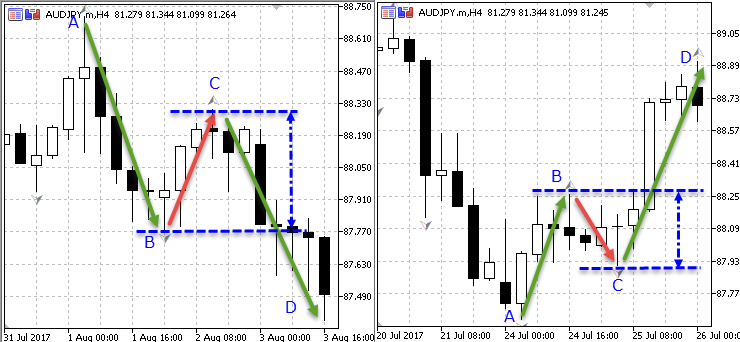Fig. 3. Movement continuation model on AUDJPY H4

In some cases, the price may break through point В and go back to position opening area. This situation cannot be considered as a trading one. This is the second check the program should conduct. Finally, in order to avoid multiple created positions, we need to introduce the limitation: 1 model — 1 one open position. This is the third check the program should perform.

1. Forming entry point control in the position opening area

Here all is simple: for a sell model, bid price should exceed correction movement low (point В). For a buy model, bid price should be lower than correction movement high (point В).

```//+------------------------------------------------------------------------+
//| 5.1 Forming entry point control in the position opening area (start)   |
//+------------------------------------------------------------------------+
//--- for a downtrend
bool First_downtrend_control_bool=(bid>=Low_base_tf[Low_Corr_wave_downtrend_base_tf]);
//--- for an uptrend
bool First_uptrend_control_bool=(bid<=High_base_tf[High_Corr_wave_uptrend_base_tf]);
//+------------------------------------------------------------------------+
//| 5.1 Forming entry point control in the position opening area (end)     |
//+------------------------------------------------------------------------+```

2. Control of a price roll-back to the position opening area

To implement this control, we should define the bar with the lowest 'low' value (for sells) or the bar with the highest 'high' value (for buys) starting with the current index and up to high/low bar of the correction movement (point В). To achieve this, the ArrayMinimum() function is used for the sell model and the ArrayMaximum() function for the buy model.

Further on, the indices are compared, the low/high index of the correction movement (point В) and the indices obtained by the ArrayMinimum() and ArrayMaximum() functions. If they match, there has been no low/high breakthrough of the correction movement, and the entire case can be considered between a trading one. If the indices do not coincide, the movement has started earlier, and it is too late to open a position.

```//+------------------------------------------------------------------------------+
//| 5.2 Control of a price roll-back to the position opening area (start)        |
//+------------------------------------------------------------------------------+
//--- for a downtrend
//find the bar with the lowest price between the bar 0 and low of the correction movement
int Second_downtrend_control_int=ArrayMinimum(Low_base_tf,0,Low_Corr_wave_downtrend_base_tf+1);
//if current bar's low is below the correction movement's low
if(Low_base_tf_0<Low_base_tf[Second_downtrend_control_int])
{
Second_downtrend_control_int=0; //this means the minimum is on bar 0
}
//if the bar with the lowest price and correction movement low match, this is the same bar
//this means the price has not moved beyond position opening area
bool Second_downtrend_control_bool=(Second_downtrend_control_int==Low_Corr_wave_downtrend_base_tf);
//---
//--- for an uptrend
//find the bar with the highest price between the bar 0 and high of the correction movement
int Second_uptrend_control_int=ArrayMaximum(High_base_tf,0,High_Corr_wave_uptrend_base_tf+1);
//if current bar's high exceeds correction movement's high
if(High_base_tf_0>High_base_tf[Second_uptrend_control_int])
{
Second_uptrend_control_int=0;//this means maximum on bar 0
}
//if the bar with the highest price and correction movement high match, this is the same bar
//this means the price has not moved beyond position opening area
bool Second_uptrend_control_bool=(Second_uptrend_control_int==High_Corr_wave_uptrend_base_tf);
//+-----------------------------------------------------------------------------+
//| 5.2 Control of a price roll-back to the position opening area (end)         |
//+-----------------------------------------------------------------------------+```

3. Elimination of duplicating positions within a single model

This control is used to limit the number of opened positions. The idea behind it: one model — one open position. Open positions are analyzed one by one. If a position is opened on the current chart, the extremum bar nearest to that position from the entry point is defined - correction movement high/low (point С from the entry point) depending on the trade type.

After that, the time of the detected bar — correction movement high/low (point С from the entry point) — is compared with the time of the current correction movement high/low (current point С). If they match, no position should be opened, since there is no position adhering to this model.

Creating sells control:

```//+---------------------------------------------------------------------------+
//| 5.3.1 For selling (start)                                                 |
//+---------------------------------------------------------------------------+
//--- declare variables
int Bar_sell_base_tf,High_Corr_wave_downtrend_base_tf_sell;
bool Third_downtrend_control_bool=false;
//--- iterate over open positions
if(PositionsTotal()>0)
{
for(i=0;i<=PositionsTotal();i++)
{
if(PositionGetTicket(i))
{
//--- define position symbol, time and type
P_symbol=string(PositionGetString(POSITION_SYMBOL));
P_type=int(PositionGetInteger(POSITION_TYPE));
P_opentime=int(PositionGetInteger(POSITION_TIME));
//--- if a position symbol matches the current chart and the trade type is "sell"
if(P_symbol==Symbol() && P_type==1)
{
//--- find the bar the position has been opened at
Bar_sell_base_tf=iBarShift(Symbol(),base_tf,P_opentime);
//--- search for correction movement high from it
//if the position has been opened on the current bar,
if(Bar_sell_base_tf==0)
{
//and the current bar is an extremum
if(High_base_tf_0[Bar_sell_base_tf]>High_base_tf[Bar_sell_base_tf+1] && High_base_tf_0[Bar_sell_base_tf]>High_base_tf[Bar_sell_base_tf+2])
{
High_Corr_wave_downtrend_base_tf_sell=Bar_sell_base_tf;//correction movement high is equal to the current bar
}
else
{
//if the current bar is not an extremum, launch the loop for searching for an extremum
for(n=Bar_sell_base_tf; n<(bars_base_tf);n++)
{
if(High_base_tf[n]>High_base_tf[n+1] && High_base_tf[n]>High_base_tf[n+2])//if the extremum is found
break;//break the loop
}
High_Corr_wave_downtrend_base_tf_sell=n;
}
//--- describe control conditions
Third_downtrend_control_bool=(
/*1. Time of the correction movement high found from position opening
matches the time of the current correction movement high*/Time_base_tf[High_Corr_wave_downtrend_base_tf_sell]==High_Corr_wave_downtrend_base_tf_time
);
}
//--- if position is opened not on the current bar
if(Bar_sell_base_tf!=0 && Bar_sell_base_tf!=1000)
{
//--- launch the loop for detecting the extremum bar
for(n=Bar_sell_base_tf; n<(bars_base_tf);n++)
{
//--- if extremum is found
if(High_base_tf[n]>High_base_tf[n+1] && High_base_tf[n]>High_base_tf[n+2])
break;//break the loop
}
High_Corr_wave_downtrend_base_tf_sell=n;
}
Third_downtrend_control_bool=(
/*1. Time of the correction movement high found from position opening
matches the time of the current correction movement high*/Time_base_tf[High_Corr_wave_downtrend_base_tf_sell]==High_Corr_wave_downtrend_base_tf_time
);
}
}
}
}
//+---------------------------------------------------------------------------+
//| 5.3.1 For selling (end)                                                   |
//+---------------------------------------------------------------------------+```
```//+---------------------------------------------------------------------------+
//| 5.3.2 For buying (start)                                                  |
//+---------------------------------------------------------------------------+
//--- declare variables
bool Third_uptrend_control_bool=false;
//--- iterate over open positions
if(PositionsTotal()>0)
{
for(i=0;i<=PositionsTotal();i++)
{
if(PositionGetTicket(i))
{
//define position symbol, type and time
P_symbol=string(PositionGetString(POSITION_SYMBOL));
P_type=int(PositionGetInteger(POSITION_TYPE));
P_opentime=int(PositionGetInteger(POSITION_TIME));
//if a position symbol coincides with the current chart and buy trade type
if(P_symbol==Symbol() && P_type==0)
{
//find the bar the position has been opened at
//search for correction movement low from it
//if the position has been opened on the current bar,
{
//and the current bar is an extremum
{
}
else
{
//if the current bar is not an extremum, launch the loop for searching for an extremum
{
if(Low_base_tf[n]<Low_base_tf[n+1] && Low_base_tf[n]<Low_base_tf[n+2])//if the extremum is found
break;//break the loop
}
}
//--- describe control conditions
Third_uptrend_control_bool=(
/*1. Time of the correction movement low found from position opening
matches the time of the current correction movement low*/Time_base_tf[Low_Corr_wave_uptrend_base_tf_buy]==Low_Corr_wave_uptrend_base_tf_time
);
}
//--- if position is opened not on the current bar
{
//--- launch the loop for detecting the extremum bar
{
//--- if extremum is found
if(Low_base_tf[n]<Low_base_tf[n+1] && Low_base_tf[n]<Low_base_tf[n+2])
break;//break the loop
}
}
//--- describe control conditions
Third_uptrend_control_bool=(
/*1. Time of the correction movement low found from position opening
matches the time of the current correction movement low*/Time_base_tf[Low_Corr_wave_uptrend_base_tf_buy]==Low_Corr_wave_uptrend_base_tf_time
);
}
}
}
}
//+---------------------------------------------------------------------------+
//| 5.3.2 For selling (end)                                                   |
//+---------------------------------------------------------------------------+```

### 7. Describing market entry conditions

The entry point should be defined on the working period — work_tf. This is necessary for timely entry into the market and, if possible, reducing the amount of risk in points. Parabolic indicator readings are used as a signal: if the indicator value on the current bar exceeds the current bar's high, while on the previous bar, the indicator value is lower than the same bar's low, then it is time to sell. For buying, the case is reversed.

```//+------------------------------------------------------------------+
//| 6. Describing market entry conditions (start)                    |
//+------------------------------------------------------------------+
//--- for selling
bool PointSell_work_tf_bool=(
/*1. Bar 1 low exceeds iSar*/Low_work_tf>Sar_array_work_tf &&
/*2. Bar 0 high is lower than iSar*/High_work_tf_0<Sar_array_work_tf_0
);
/*1. Bar 1 high is below iSar*/High_work_tf<Sar_array_work_tf &&
/*2. Bar 0 low exceeds iSar*/Low_work_tf_0>Sar_array_work_tf_0
);
//+------------------------------------------------------------------+
//| 6. Describing market entry conditions (end)                      |
//+------------------------------------------------------------------+```

At this stage, we combine all previously created conditions and controls into a single logic variable.

```//+------------------------------------------------------------------+
//| 7. Describing trading conditions (start)                         |
//+------------------------------------------------------------------+
//--- for selling
bool OpenSell=(
/*1. model formed*/Model_downtrend_base_tf==true &&
/*2. control 1 allows opening a position*/First_downtrend_control_bool==true &&
/*3. control 2 allows opening a position*/Second_downtrend_control_bool==true &&
/*4. control 3 allows opening a position*/Third_downtrend_control_bool==false &&
/*5. Entry point to work_tf*/PointSell_work_tf_bool==true
);
//--- for selling
/*1. model formed*/Model_uptrend_base_tf==true &&
/*2. control 1 allows opening a position*/First_uptrend_control_bool==true &&
/*3. control 2 allows opening a position*/Second_uptrend_control_bool==true &&
/*4. control 3 allows opening a position*/Third_uptrend_control_bool==false &&
);
//+------------------------------------------------------------------+
//| 7. Describing trading conditions (end)                           |
//+------------------------------------------------------------------+```

### 9. Working with trading operations

Working with trading operations can be divided into:

• Setting positions;
• Setting a take profit;
• Moving a position to a breakeven.

1. Setting positions

```//+------------------------------------------------------------------+
//| 8. Working with trading operations (start)                       |
//+------------------------------------------------------------------+
//--- define stop loss levels
//+------------------------------------------------------------------+
//| 8.1 Setting positions (start)                                    |
//+------------------------------------------------------------------+
//--- for selling
if(OpenSell==true)
{
RiskSize_points=(SLPrice_sell-bid)*f;//define sl in points as integer
if(RiskSize_points==0)//zero divide check
{
RiskSize_points=1;
}
CostOfPoint_position=SummRisk/RiskSize_points;//define position price in points considering sl
Lot=CostOfPoint_position/CostOfPoint;//calculate lot for opening a position
//open a position
}
{
RiskSize_points=(bid-SLPrice_buy)*f;//define sl in points as integer
if(RiskSize_points==0)//zero divide check
{
RiskSize_points=1;
}
CostOfPoint_position=SummRisk/RiskSize_points;//define position price in points considering sl
Lot=CostOfPoint_position/CostOfPoint;//calculate lot for opening a position
//open a position
}
//+------------------------------------------------------------------+
//| 8.1 Setting positions (end)                                      |
//+------------------------------------------------------------------+```

2. Setting a take profit

```//+------------------------------------------------------------------+
//| 8.2 Setting take profit (start)                                  |
//+------------------------------------------------------------------+
if(TP_mode==true)
{
if(PositionsTotal()>0)
{
for(i=0;i<=PositionsTotal();i++)
{
if(PositionGetTicket(i))
{
//get position values
SL_double=double (PositionGetDouble(POSITION_SL));
OP_double=double (PositionGetDouble(POSITION_PRICE_OPEN));
TP_double=double (PositionGetDouble(POSITION_TP));
P_symbol=string(PositionGetString(POSITION_SYMBOL));
P_type=int(PositionGetInteger(POSITION_TYPE));
P_profit=double (PositionGetDouble(POSITION_PROFIT));
P_ticket=int (PositionGetInteger(POSITION_TICKET));
P_opentime=int(PositionGetInteger(POSITION_TIME));
if(P_symbol==Symbol())
{
if(P_type==0 && TP_double==0)
{
double TP_size_buy=SL_size_buy*M;//multiply sl by the ratio of the one set in the inputs
//modify a position
}
if(P_type==1 && TP_double==0)
{
double SL_size_sell=SL_double-OP_double;//define sl in points
double TP_size_sell=SL_size_sell*M;//multiply sl by the ratio of the one set in the inputs
double TP_price_sell=OP_double-TP_size_sell;//define tp level
//modify a position
}
}
}
}
}
}
//+------------------------------------------------------------------+
//| 8.2 Set take profit (end)                                        |
//+------------------------------------------------------------------+```

3. Moving position to a breakeven

```//+------------------------------------------------------------------+
//| 8.3 Moving a position to a breakeven (start)                     |
//+------------------------------------------------------------------+
double Size_Summ=breakeven*SummRisk;//define profit level, after which a position should be moved to a breakeven
if(Breakeven_mode==true && breakeven!=0)
{
if(PositionsTotal()>0)
{
for(i=0;i<=PositionsTotal();i++)
{
if(PositionGetTicket(i))
{
//get position values
SL_double=double (PositionGetDouble(POSITION_SL));
OP_double=double (PositionGetDouble(POSITION_PRICE_OPEN));
TP_double=double (PositionGetDouble(POSITION_TP));
P_symbol=string(PositionGetString(POSITION_SYMBOL));
P_type=int(PositionGetInteger(POSITION_TYPE));
P_profit=double (PositionGetDouble(POSITION_PROFIT));
P_ticket=int (PositionGetInteger(POSITION_TICKET));
P_opentime=int(PositionGetInteger(POSITION_TIME));
if(P_symbol==Symbol())
{
if(P_type==0 && P_profit>=Size_Summ && SL_double<OP_double)
{
}
if(P_type==1 && P_profit>=Size_Summ && SL_double>OP_double)
{
}
}
}
}
}
}
//+------------------------------------------------------------------+
//| 8.3 Moving a position to a breakeven (end)                       |
//+------------------------------------------------------------------+
//+------------------------------------------------------------------+
//| 8. Working with trading operations (end)                         |
//+------------------------------------------------------------------+```

### 5. Collecting statistical data

First you need to decide on a set of indicators for statistics:

1. Symbol;
2. Deal type;
3. Entry time;
4. Open price;
5. Stop loss level;
6. Stop loss size;
7. Maximum profit level;
8. Maximum profit size;
9. Deal duration.

It is necessary to make the assumption that the maximum profit point is the high/low of the first upper/lower fractal on the main period formed after the bar the position was opened at.

First, we need to test the EA operation in the strategy tester. For a test, I have selected AUDJPY for the period of 01.01.2018—29.08.2018. D1 was selected as the main period, while H6 was used as a working timeframe. Risk per deal — \$100. Position moving to a breakeven 1/2, setting take profit — 1/3 (risk/profit).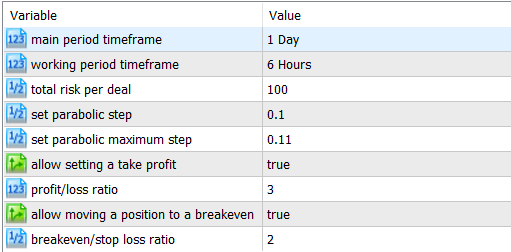Fig. 4. EA inputs

After testing, save the report in the CSV file. In the terminal local folder, create the new report.csv file. Copy report data to it (from the Order section). We should delete the lines related to position closing as shown in Figure 5: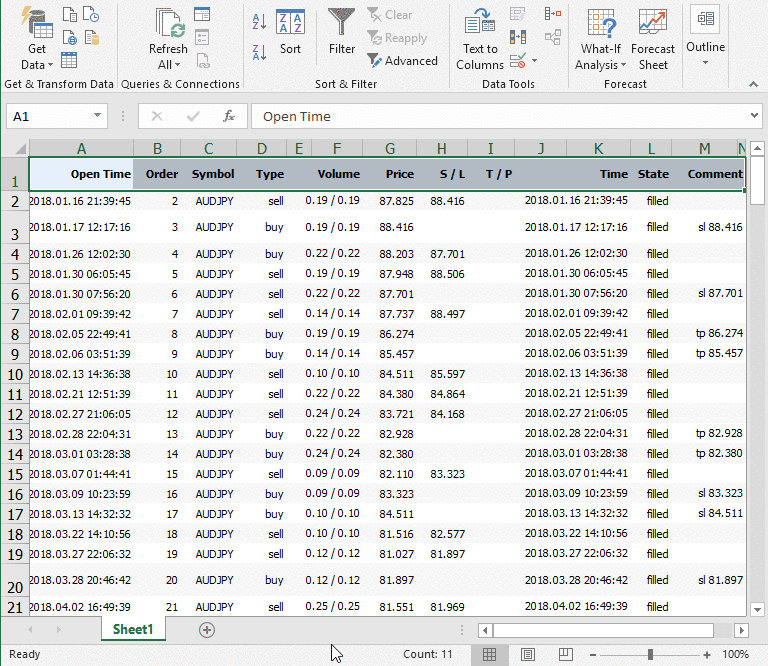Fig. 5. Deleting lines related to position closing from the report

The columns to be copied:

1. Open time;
2. Symbol;
3. Type;
4. Price;
5. S/L.

As a result, the report.csv file will look like this: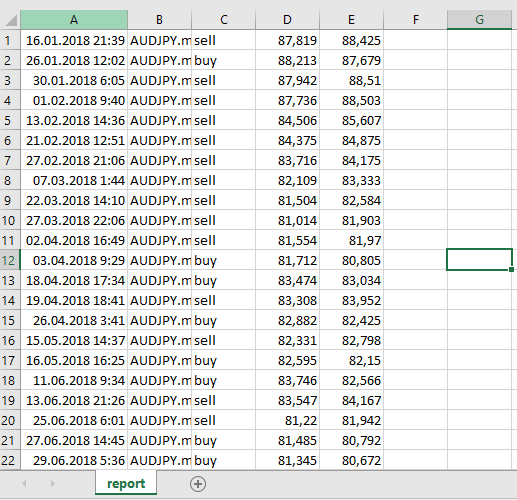Fig. 6. report.csv file contents

Now, we need to create a script that reads data from the report.csv file and creates the new file_stat.csv file with an additional statistical info:

1. SL size;
2. Maximum profit level;
3. Maximum profit size;
4. Deal duration in bars.

To solve this task, I used a ready-made solution from the "Reading a file with separators to an array" section of the "MQL5 Programming Basics: Files" article. I also added the arrays and their filling for storing the column values in the file_stat.csv file.

Create a new script and write the code of the function for reading files to the array under the OnStart() function:

```//+------------------------------------------------------------------+
//| Reading to array function (start)                                |
//+------------------------------------------------------------------+
{
ResetLastError();
if(h==INVALID_HANDLE)
{
int ErrNum=GetLastError();
printf("File open error %s # %i",FileName,ErrNum);
return(false);
}
int lcnt=0; // variable for calculating strings
int fcnt=0; // variable for calculating string fields
while(!FileIsEnding(h))
{
// new string (new structure array element)
if(lcnt>=ArraySize(Lines))
{ // structure array fully filled
ArrayResize(Lines,ArraySize(Lines)+1024); // increase the array size by 1024 elements
}
ArrayResize(Lines[lcnt].field,64);// change the array size in the structure
Lines[lcnt].field=str; // assign the value of the first field
// start reading remaining fields in a string
fcnt=1; // while one element in the field array is occupied
while(!FileIsLineEnding(h))
{ // read the remaining fields in a string
if(fcnt>=ArraySize(Lines[lcnt].field))
{ // array of fields is completely filled
ArrayResize(Lines[lcnt].field,ArraySize(Lines[lcnt].field)+64); // increase the array size by 64 elements
}
Lines[lcnt].field[fcnt]=str; // assign the value of the next field
fcnt++; // increase the field counter
}
ArrayResize(Lines[lcnt].field,fcnt); // change field array size according to the actual number of fields
lcnt++; // increase the string counter
}
ArrayResize(Lines,lcnt); // change the array of structures (strings) according to the actual number of strings
FileClose(h);
return(true);
}
//+------------------------------------------------------------------+
//| Reading to array function (end)                                  |
//+------------------------------------------------------------------+```

Next, specify the inputs:

```#property script_show_inputs
//--- inputs
input ENUM_TIMEFRAMES base_tf;  //base period timeframe
input double sar_step=0.1;      //set parabolic step
input double maximum_step=0.11; //set parabolic maximum step
//--- declare variables for indicator handles
int Fractal_base_tf;             //iFractal indicator handle
//--- declare variables for base_tf
double High_base_tf[],Low_base_tf[];             //arrays for storing the prices of High and Low bars
double FractalDown_base_tf[],FractalUp_base_tf[];//array for storing the prices of the iFractall indicator
//--- array structure
struct SLine
{
string            field[];
};```

Inside the OnStart() function, get the iFractals indicator handle, as well as declare and fill the High/Low prices array. We also need the bars_base_tf variable to be used in the for loop and the f variable to store the price digit capacity depending on the number of decimal places in the symbol price. This variable is used for converting stop loss and maximum profit values into integers.

```//--- get iFractal indicator handles
Fractal_base_tf=iFractals(Symbol(),base_tf);
//--- set the order of arrays as in the timeseries for base_tf
ArraySetAsSeries(High_base_tf,true);
ArraySetAsSeries(Low_base_tf,true);
ArraySetAsSeries(FractalDown_base_tf,true);
ArraySetAsSeries(FractalUp_base_tf,true);
//--- initial filling of arrays for base_tf
CopyHigh(Symbol(),base_tf,0,1000,High_base_tf);
CopyLow(Symbol(),base_tf,0,1000,Low_base_tf);
CopyBuffer(Fractal_base_tf,0,TimeCurrent(),1000,FractalUp_base_tf);
CopyBuffer(Fractal_base_tf,1,TimeCurrent(),1000,FractalDown_base_tf);
//--- variables for storing data on the number of bars
int bars_base_tf=Bars(Symbol(),base_tf);
//number of decimal places in the symbol price
int Digit=(int)SymbolInfoInteger(_Symbol,SYMBOL_DIGITS);
//define the current symbol's price capacity
double f=1;
if(Digit==5) {f=100000;}
if(Digit==4) {f=10000;}
if(Digit==3) {f=1000;}
if(Digit==2) {f=100;}
if(Digit==1) {f=10;}```

Next, declare arrays and variables:

```//--- declare variables and arrays
int i,j,n; //variables for loops
datetime opentime[];//array for storing position setting time
string symbol[];//array for storing symbols
string type[];////array for storing deal types
string openprice[];//array for storing open prices
string  sl_price[];//array for storing stop loss level
int index[];//array for storing the index of the bar position was set at
int down_fractal[];//array for storing lower fractal indices
int up_fractal[];//array for storing upper fractal indices
double sl_size_points[];//array for storing stop loss size in points
string maxprofit_price[];//array for storing maximum profit levels
double maxprofit_size_points[];//array for storing maximum profit value
int duration[];//array for storing data on the wave duration in bars
bool maxprofit_bool[];//array that ensures the position is not activated by sl
int maxprofit_int[];//array for defining the minimum/maximum bar. It is to be used with maxprofit_bool[]```

After this, move on to reading the data from the file to the arrays:

```   SLine lines[];
int size=0;
{
Alert("Error, see details in the \"Experts\"" tab);
}
else
{
size=ArraySize(lines);
ArrayResize(opentime,ArraySize(lines));
ArrayResize(symbol,ArraySize(lines));
ArrayResize(type,ArraySize(lines));
ArrayResize(openprice,ArraySize(lines));
ArrayResize(sl_price,ArraySize(lines));
ArrayResize(index,ArraySize(lines));
ArrayResize(down_fractal,ArraySize(lines));
ArrayResize(up_fractal,ArraySize(lines));
ArrayResize(sl_size_points,ArraySize(lines));
ArrayResize(maxprofit_price,ArraySize(lines));
ArrayResize(maxprofit_size_points,ArraySize(lines));
ArrayResize(duration,ArraySize(lines));
ArrayResize(maxprofit_bool,ArraySize(lines));
ArrayResize(maxprofit_int,ArraySize(lines));
for(i=0;i<size;i++)
{
for(j=0;j<ArraySize(lines[i].field);j=j+5)//select fields by position open time column
{
opentime[i]=(datetime)(lines[i].field[j]);//write data to array
}
for(j=1;j<ArraySize(lines[i].field);j=j+4)//select fields by symbol column
{
symbol[i]=(lines[i].field[j]);//write data to array
}
for(j=2;j<ArraySize(lines[i].field);j=j+3)//select fields by deal type column
{
type[i]=(lines[i].field[j]);//write data to array
}
for(j=3;j<ArraySize(lines[i].field);j=j+2)//select fields by open price column
{
openprice[i]=(lines[i].field[j]);//write data to array
}
for(j=4;j<ArraySize(lines[i].field);j=j+1)//select fields by stop loss column
{
sl_price[i]=(lines[i].field[j]);//write data to array
}
}
}
//-----------------------------------------------------```

The openrpice[] and sl_price[] arrays have a string data type. To use them in calculations, convert them to double type using the StringToDouble() function. However, the decimals are lost in this case. To avoid this, use the StringReplace() function to replace the comma with the period:

```   for(i=0;i<size;i++)
{
StringReplace(openprice[i],",",".");
StringReplace(sl_price[i],",",".");
}```

Then define the indices of the bars positions have been placed at:

```//--- define indices of the bars positions were opened at
for(i=0;i<size;i++)
{
index[i]=iBarShift(Symbol(),PERIOD_D1,opentime[i]);//write data to array
}```

After that, find lower and upper fractals closest to placed positions:

```//--- look for a down fractal for selling
for(i=0;i<size;i++)
{
if(type[i]=="sell")
{
for(n=index[i];n>0;n--)
{
if(FractalDown_base_tf[n]!=EMPTY_VALUE)
break;
}
down_fractal[i]=n;
}
}
//--- look for an up fractal for buying
for(i=0;i<size;i++)
{
{
for(n=index[i];n>0;n--)
{
if(FractalUp_base_tf[n]!=EMPTY_VALUE)
break;
}
up_fractal[i]=n;
}
}```

Next, define the stop loss in points and convert the number of points into integer:

```//--- stop loss in points
for(i=0;i<size;i++)
{
if(type[i]=="sell")
{
sl_size_points[i]=(StringToDouble(sl_price[i])-StringToDouble(openprice[i]))*f;
}
{
sl_size_points[i]=(StringToDouble(openprice[i])-StringToDouble(sl_price[i]))*f;
}
}```

Based on the previously detected fractals, you can determine the maximum profit level. But first we need to ensure that the position will not be prematurely closed by stop loss. Check code:

```//--- make sure the position is not closed by sl before reaching the maximum profit
//--- for sell deals
for(i=0;i<size;i++)
{
if(type[i]=="sell")
{
for(n=index[i];n>down_fractal[i];n--)
{
if(High_base_tf[n]>=StringToDouble(sl_price[i]))
break;
}
maxprofit_int[i]=n;
maxprofit_bool[i]=(n==down_fractal[i]);
}
}
for(i=0;i<size;i++)
{
{
for(n=index[i];n>up_fractal[i];n--)
{
if(Low_base_tf[n]<=StringToDouble(sl_price[i]))
break;
}
maxprofit_int[i]=n;
maxprofit_bool[i]=(n==up_fractal[i]);
}
}```

Now you can write the code for determining the maximum profit level:

```//--- maximum profit level
for(i=0;i<size;i++)
{
if(type[i]=="sell" && maxprofit_bool[i]==true)
{
maxprofit_price[i]=(string)Low_base_tf[down_fractal[i]];
}
if(type[i]=="sell" && maxprofit_bool[i]==false)
{
maxprofit_price[i]="";
}
{
maxprofit_price[i]=(string)High_base_tf[up_fractal[i]];
}
{
maxprofit_price[i]="";
}
}```

Then you can determine the size of the maximum profit. The profit will be negative by stop loss if the control is activated:

```   for(i=0;i<size;i++)
{
if(type[i]=="sell" && maxprofit_bool[i]==true)
{
maxprofit_size_points[i]=(StringToDouble(openprice[i])-Low_base_tf[down_fractal[i]])*f;
}
if(type[i]=="sell" && maxprofit_bool[i]==false)
{
maxprofit_size_points[i]=sl_size_points[i]*-1;
}
{
maxprofit_size_points[i]=(High_base_tf[up_fractal[i]]-StringToDouble(openprice[i]))*f;
}
{
maxprofit_size_points[i]=sl_size_points[i]*-1;
}
}```

Finally, let's define the duration between the bar the position is placed at and the maximum profit one (in bars). If sl closing control is activated, the duration is defined as a difference between the bar the position is set at and the one, at which sl is triggered:

```//--- calculate deal duration in bars
for(i=0;i<size;i++)
{
if(type[i]=="sell" && maxprofit_bool[i]==true)
{
duration[i]=index[i]-(int)down_fractal[i];
}
if(type[i]=="sell" && maxprofit_bool[i]==false)
{
duration[i]=index[i]-maxprofit_int[i];
}
{
duration[i]=index[i]-(int)up_fractal[i];
}
{
duration[i]=index[i]-maxprofit_int[i];
}
}```

After that, let's replace periods back to commas for correct display of the parameters:

```   for(i=0;i<size;i++)
{
StringReplace(openprice[i],".",",");
StringReplace(sl_price[i],".",",");
StringReplace(maxprofit_price[i],".",",");
}```

Now, it only remains to write the obtained data to the file_stat.csv file:

```//--- write the data to the new statistics file
//--- check for opening
if(h==INVALID_HANDLE)
{
return;
}
else
{
FileWrite(h,
/*1 symbol*/"Symbol",
/*2 deal type*/"Deal type",
/*3 entry time*/"Open time",
/*4 open price*/"Open price",
/*5 sl level*/"SL",
/*6 sl level*/"SL size",
/*7 max profit level*/"Max profit level",
/*8 max profit value*/"Max profit value",
/*9 duration*/"Duration in bars");
//--- move to the end
FileSeek(h,0,SEEK_END);
for(i=0;i<size;i++)
{
FileWrite(h,
/*1 symbol*/symbol[i],
/*2 deal type*/type[i],
/*3 entry time*/TimeToString(opentime[i]),
/*4 open price*/openprice[i],
/*5 sl level*/sl_price[i],
/*6 sl size*/NormalizeDouble(sl_size_points[i],2),
/*7 max profit level*/maxprofit_price[i],
/*8 max profit size*/NormalizeDouble(maxprofit_size_points[i],2),
/*9 duration*/duration[i]);
}
}
FileClose(h);

Check: launch the script on the chart after setting the base timeframe period in the inputs (which is D1 in my case). After that, the new file_stat.csv file with the following set of parameters appears in the terminal's local folder: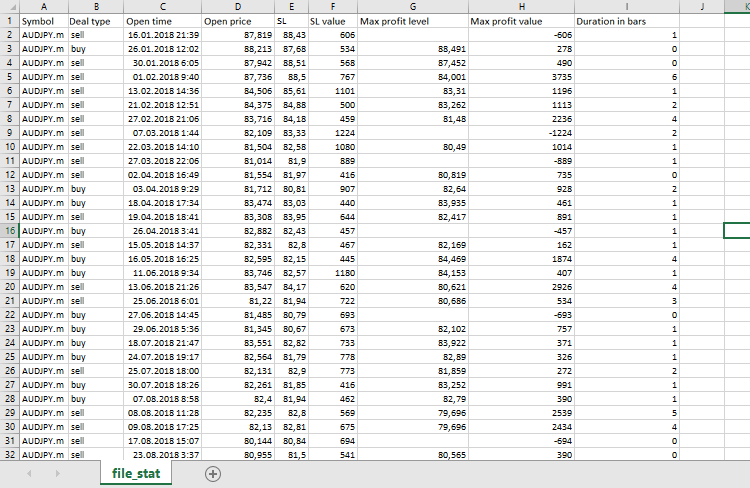Fig. 7. file_stat.csv file contents

### 6. Conclusion

In this article, we have analyzed the method of programmatically determining one of the movement continuation models. The key idea of the method is a search for a correction movement high/low extremum without applying any indicators. The consecutive points of the model are then detected based on the found extremum.

We also discussed the method of collecting statistical data based on the results of testing in the strategy tester by writing the test results into an array and their subsequent processing. I believe, it is possible to develop a more efficient way of collecting and processing statistical data. However, this method seems most simple and comprehensive to me.

Keep in mind that the article describes the minimum requirements for defining the model, and most importantly, the most minimal set of controls provided by the EA. For real trading, the set of controls should be expanded.

Below are examples of movement continuation model recognition: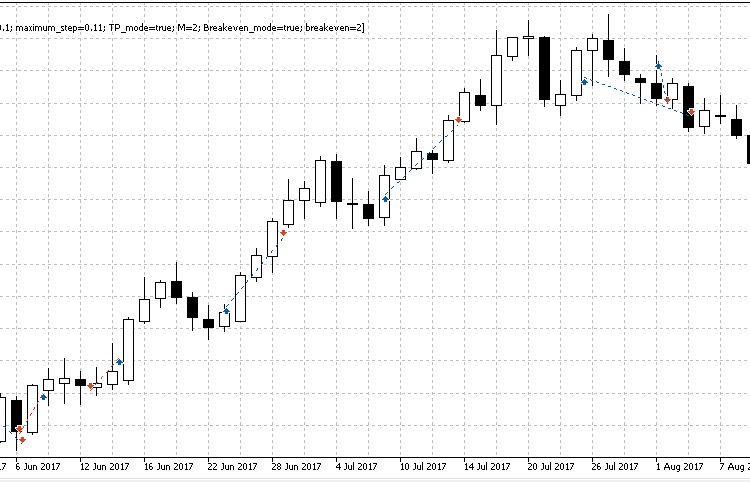Fig. 8. Sample movement continuation model recognition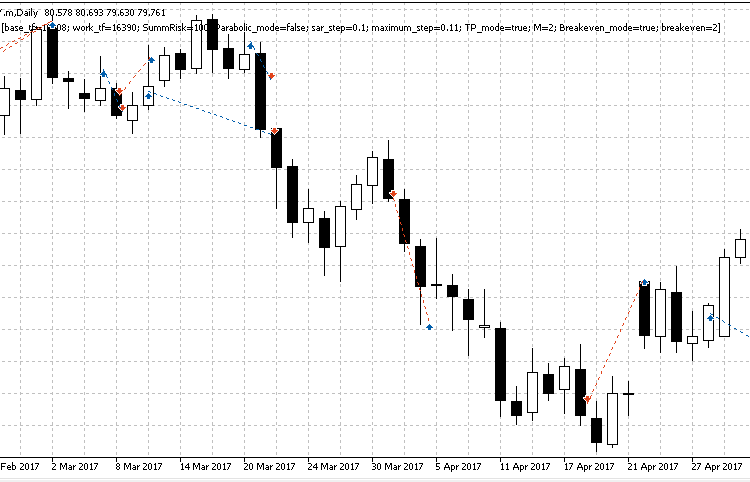Fig. 9. Sample movement continuation model recognition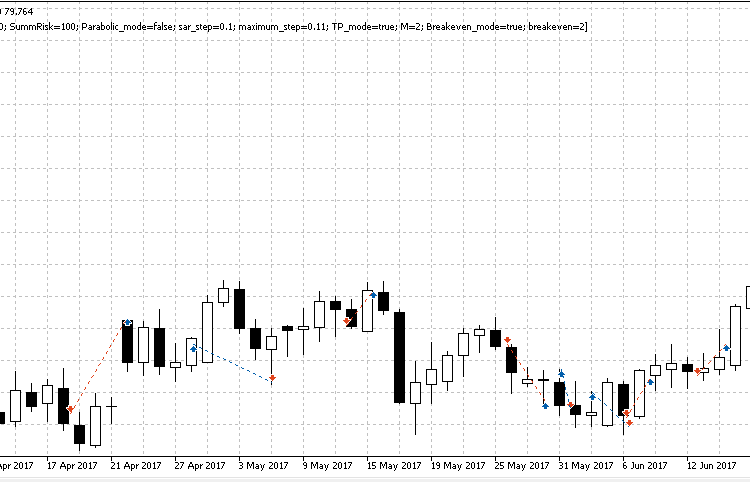Fig. 10. Sample movement continuation model recognition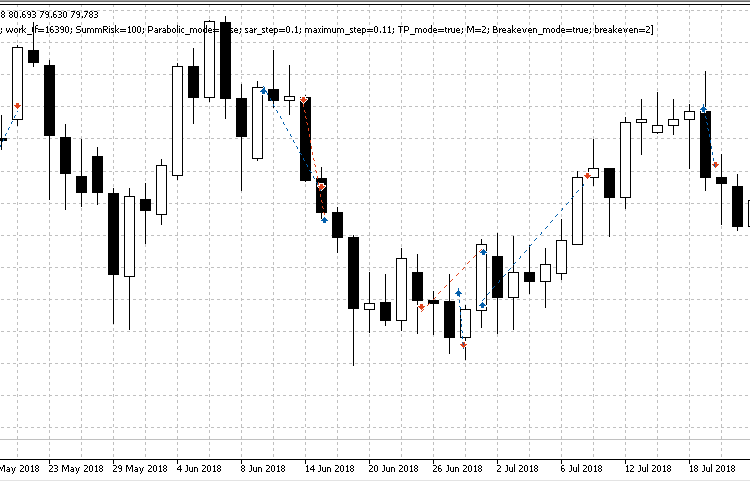Fig. 11. Sample movement continuation model recognition

### Programs used in the article

# Name Type Description
2 MQL5_POST_final Expert Advisor EA defining the movement continuation model
3 Report_read Script Script for collecting statistics

Translated from Russian by MetaQuotes Software Corp.
Original article: https://www.mql5.com/ru/articles/4222

Attached files |
MQL5.zip (128.44 KB)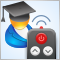EA remote control methods

The main advantage of trading robots lies in the ability to work 24 hours a day on a remote VPS server. But sometimes it is necessary to intervene in their work, while there may be no direct access to the server. Is it possible to manage EAs remotely? The article proposes one of the options for controlling EAs via external commands.100 best optimization passes (part 1). Developing optimization analyzer

The article dwells on the development of an application for selecting the best optimization passes using several possible options. The application is able to sort out the optimization results by a variety of factors. Optimization passes are always written to a database, therefore you can always select new robot parameters without re-optimization. Besides, you are able to see all optimization passes on a single chart, calculate parametric VaR ratios and build the graph of the normal distribution of passes and trading results of a certain ratio set. Besides, the graphs of some calculated ratios are built dynamically beginning with the optimization start (or from a selected date to another selected date).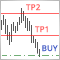Using limit orders instead of Take Profit without changing the EA's original code

Using limit orders instead of conventional take profits has long been a topic of discussions on the forum. What is the advantage of this approach and how can it be implemented in your trading? In this article, I want to offer you my vision of this topic.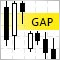Gap - a profitable strategy or 50/50?

The article dwells on gaps — significant differences between a close price of a previous timeframe and an open price of the next one, as well as on forecasting a daily bar direction. Applying the GetOpenFileName function by the system DLL is considered as well.